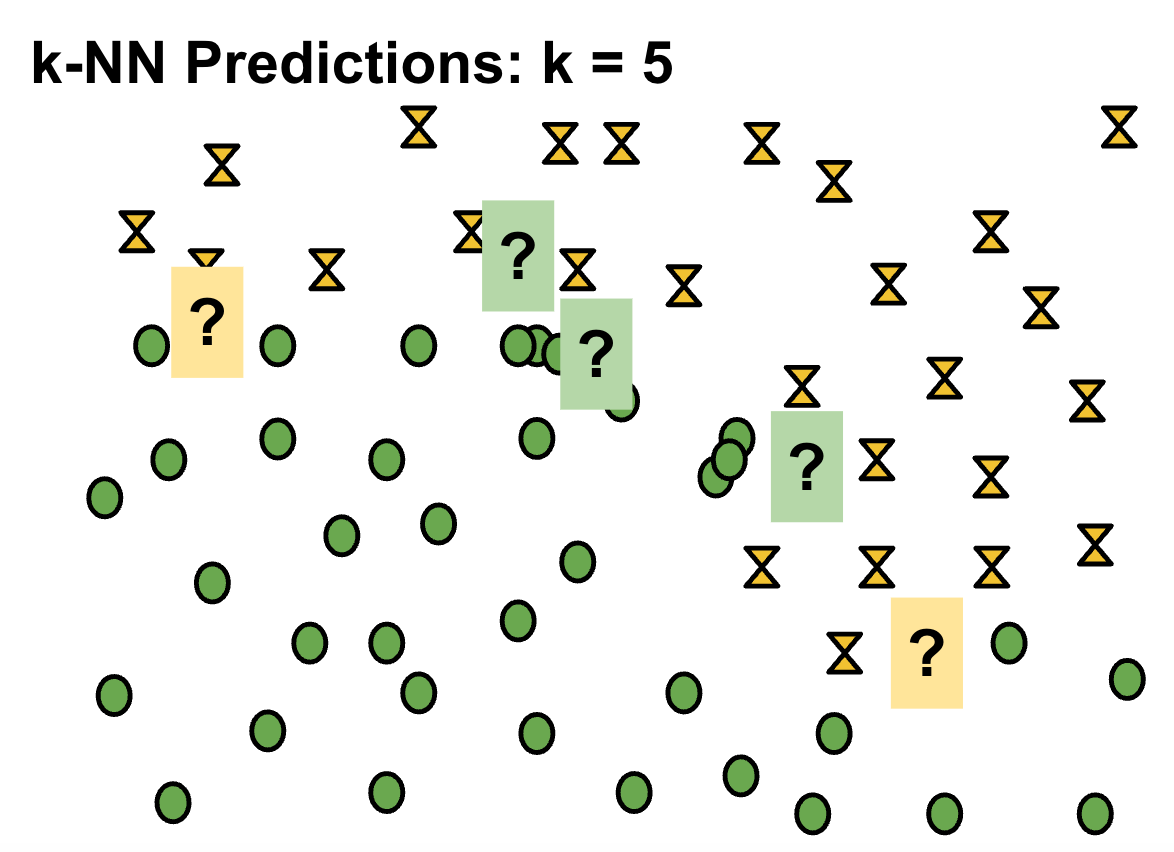# And the Winner Is…

A Clear Answer to Which Machine Learning Approach is the Best

A commonly asked question among those studying machine learning for the first time, especially after they have reviewed several different machine learning algorithms, is “Which one is the best one?” or alternatively, “How do I know which approach to use when I’m getting started with new data?”

My typical answer to such question is “I have no idea” and “nobody knows” which is just a clever way of avoiding the cliche of “it depends.” But that’s not exactly true. Indeed, there is a way to determine the best machine learning model and approach for a given data set.

You simply find the one that makes the best predictions.

I’m not trying to be difficult. It’s just the correct answer to the question. To further clarify, let’s take a look at an example.

Say you have a data set with a training label distribution that looks like this:

Now before we try to pick a modeling approach, let’s create some test data points — the data we will use to validate whether our model is good at making predictions in the real world. The question marks in the image below represent those test points:

To keep it simple, let’s assume we are trying to decide between three different modeling approaches — linear classifier, Naive Bayes, and k-Nearest Neighbors. Since linear classifiers are relatively popular, we decide to use one of them — maybe logistic regression — and we end up with a dividing hyperplane that splits the data like this for the lowest possible loss:

So far so good! Now we make our predictions based on this separating hyperplane. We will color the question marks yellow if we predict the golden X class, and green if we predict the green oval class. (We could just have easily chosen +1 and -1, it’s just less visually interesting…) So our test point predictions go like this:

Before we decide whether these are “good” predictions or not, let’s generate some predictions on the same data using k-NN. Now remember, k-NN can generate different results based on the value selected for k, so let’s generate predictions using three values — 1, 3, and 5:Interesting note: These predictions are exactly the opposite of the linear approach predictions.

And finally, we decide to generate some predictions using Naive Bayes as well, and we end up with a prediction distribution that looks like this:

So, we have now generated five different predictive models using three different modeling approaches. None of them generated the same predictions for our five test points. Which one would we deem to be the best, from a predictive standpoint?

Again — the one where the predictions the model made aligned most closely with reality.

Before we actually generate predictions and evaluate their accuracy, we have no way of determining how good a given modeling approach is going to be on a given data set. It could well be that in one scenario, Naive Bayes was the most accurate, and then in another k-NN with k=3 was the winner, and yet another might well be our linear classifier. There is simply no way to know before you actually run the tests, and anyone who tries to tell you differently is selling something.

The same is true for more complex modeling approaches like decision trees and neural networks. Yes, they can draw more complex decision boundaries and align more closely with your training data, but they can also be outperformed by more straightforward modeling approaches if the nature of reality of the question you’re trying to answer fits that better than the fancy dividing lines.

While it’s true that there are categories of problems where a particular modeling approach tends to work better than others — for example, XGBoost tends to do really well on tabular data, and various neural networking approaches tend to shine on AI vision and natural language processing tasks — it is also true that there are circumstances in which those approaches cannot avoid the overfitting problem, or reality is just noisy, or insert any anomaly here that might make a simpler model better at real-world predictions. As a data scientist, you should be inclined to at least use the simpler, more straightforward modeling approaches as a way to create a baseline of performance, and then when employing more complex modeling approaches, make sure they outperform that baseline for the particular problem you are trying to solve on your particular data set. If you assume too much going in about what approach is best, you may end up spending a lot of money and time building models that could easily have been replicated or even beaten at a fraction of the cost in a fraction of the time. Hopefully, the visual examples above help illustrate that idea.

Or, put another way: the more you realize you don’t know going in, the better at data science you will be.

--

--